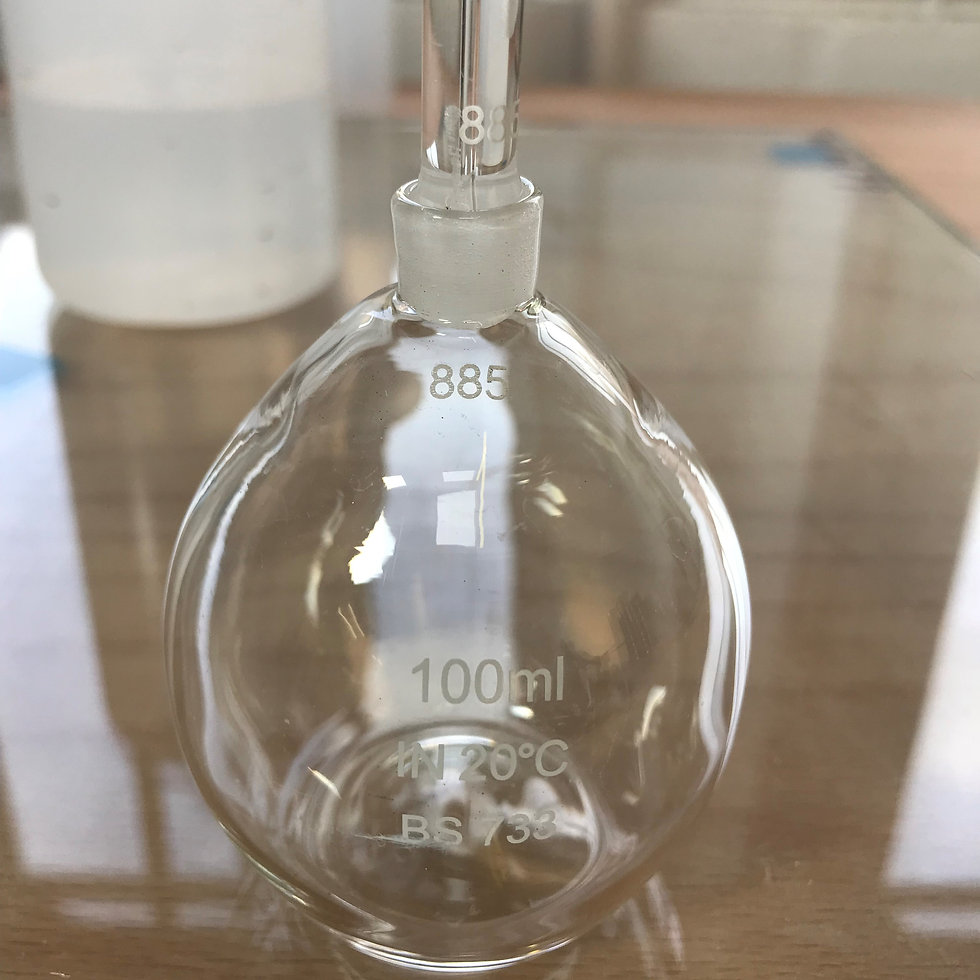## Particle Density

#### The specific gravity/particle density of soils and aggregates

Particle density of soils refers to the density of the solid particles collectively. It is expressed as the ratio of the total mass of the solid particles to their total volume, excluding pore spaces between particles.

The easiest way to understand it is to think of soil as a mix of solids (particles), water and air. Using particle density and moisture content it is possible to calculate the volume of air voids in a soil which is important in some circumstances for assessing compaction levels in earthworks as the higher the air voids then theoretically the lower the level of compaction.

## Not much use on its own

This test is ideally suited to supporting other tests where its value may be assumed, such as compaction testing.

## Various methods

Depending on the material there are various methods which we can adopt for this type of test.

## In cases of dispute

If there is a dispute over compaction levels then this test can confirm particle density assumed in any calculations of voids.